# Stochastic limit of quantum theory

The quantum-mechanical description of a physical system is achieved by assigning: i) a Hilbert space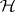, called the state space of the system; ii) a family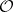of self-adjoint operators on, called the observables (cf. Self-adjoint operator); iii) a dynamical equation of the form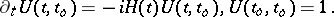(a1)

Here, for each real number(time),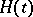is a self-adjoint operator, called the Hamiltonian (or interaction picture Hamiltonian;is the imaginary unit;denotes the identity operator on; and the derivative is meant in a topology that is left unspecified. If the mathematical model has been correctly formulated, the Cauchy problem (a1) admits a unique solution for any choice of the initial time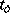and for any real number. Moreover, for any pair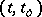, the operatoris unitary (cf. Unitary operator) and the uniqueness of the solution implies the relation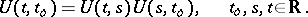(a2)

The aim of quantum theory is to compute quantities of the form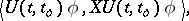(a3)

whereis a unit vector in(preparation) andis an observable (measurement). Often, this problem can be reduced to the calculation of the transition amplitudes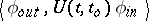(a4)

for sufficiently many vectors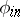(initial) and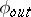(final) of.

This abstract scheme is simple, but in order to put it to work in concrete situations, i.e. to deduce from it predictions on the real world, one has to introduce approximations either to solve equation (a1) or to read the physics in its solution. The usual approximation procedures are based on perturbation theory. The stochastic limit of quantum theory is a new approximation procedure in which the fundamental laws themselves, as described by the pair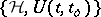(the set of observables being fixed once for all, hence left implicit), are approximated rather than the single expectation values (a3). Significant examples are obtained by considering models with Hamiltonians of the form(in this caseis called the coupling constant). The first step of the stochastic method is to rescale time in the solutionof equation (a1) according to the Friedrichs–van Hove scaling:. One then considers the limit of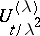asin a topology which is the quantum analogue of the probabilistic notion of convergence in law (cf. Convergence in probability; Quantum probability). Recently (1996), several different types of rescalings have begun to emerge, which also give rise to interesting stochastic limits, but for the moment the majority of results has been obtained for the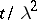-scaling. Physically, the rescaled time gives the time scale of the fast degrees of freedom and the original timethat of the slow ones, i.e. one is studying the long-time cummulative effects of the interaction. The long time (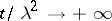) compensates the weak coupling, or low density (), and new rich structures emerge from the detailed study of several models: as usual with non-linear problems, there is no general theorem which encompasses all the results. In fact, each limiting structure strongly depends on the specific form of the non-linearity. There is, however, a general pattern which depends only on general qualitative features and this allows one to insert the multiplicity of individual results within the framework of a theory.

The remarkable new feature of this theory is that, for many models, the limit evolution operator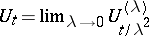not only exists, but is unitary and satisfies a quantum stochastic differential equation driven by a special type of quantum field which, in quantum optics or solid state physics, is called a quantum noise (in the recent, disjoint, literature on quantum field theory, such fields have also implicitly made their appearance and have been called master fields). The specific features of this limit field strongly depend on the model. The advantage of the new stochastic equation versus the original one is that one gets a simplification of the model in the sense that many physical effects are more easily read in the new equations. It should be emphasized that the unitarity of the evolution operator is preserved under the stochastic limit. This throws a new light on the problem of the emergence of irreversible behaviour from reversible evolutions, allowing one to go beyond the usual picture of the open system approach, based on the system-reservoir picture and the master equation. In fact, several recent results show that in several cases the stochastic limit can be performed on a single system. Physically this means that the fast degrees of freedom of a single system can act as noise on the slow ones and, in the stochastic limit, a kind of separation of these degrees of freedom takes place. In this sense one can say that the stochastic limit also provides a dynamical approach to quantum chaos.

Other applications of the stochastic limit are to stochastic bosonization in arbitrary dimensions, to the quantum components in the cosmological red shift, to the largelimit in QCD, etc.